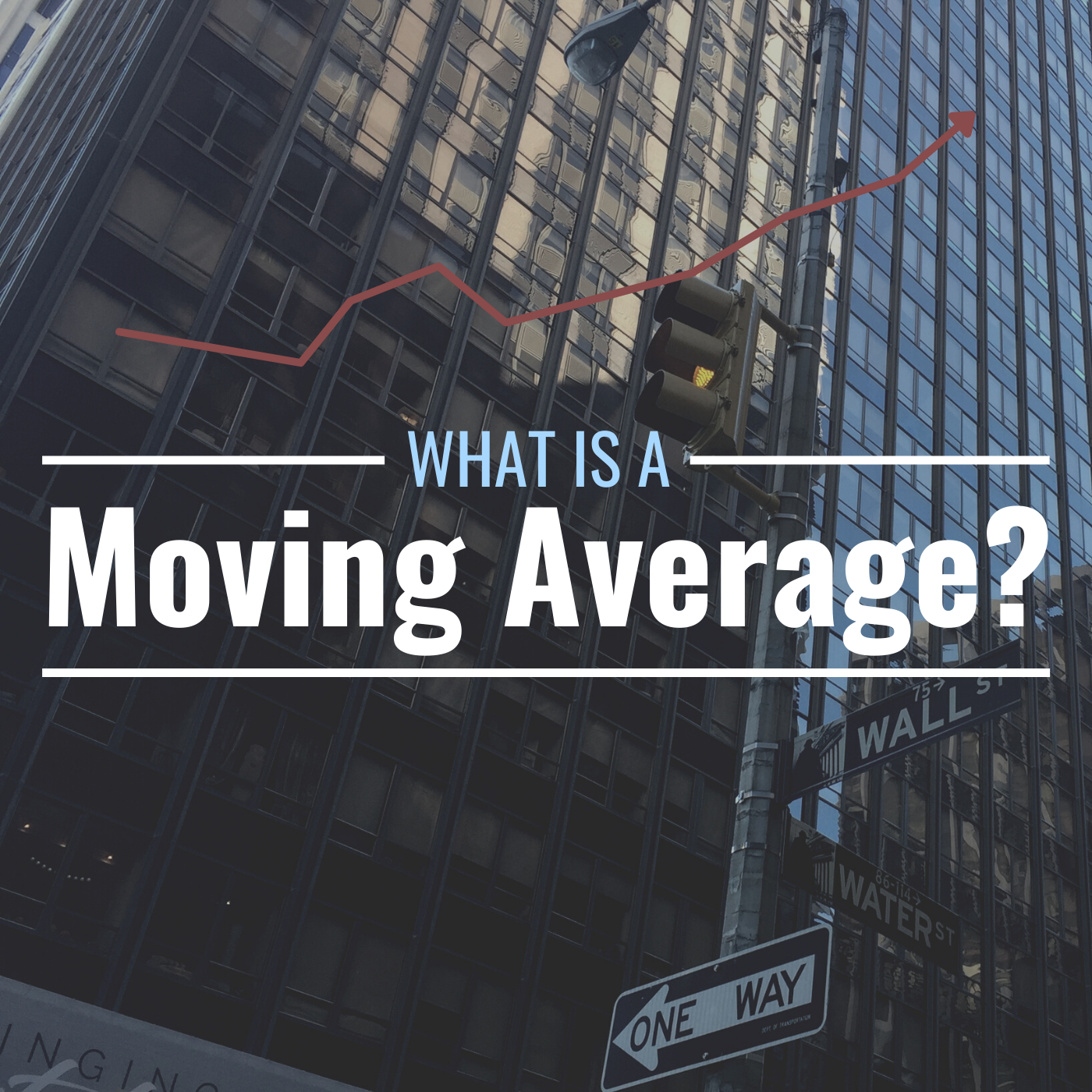# What Is a Moving Average? Definition, Calculation & Example

This technical indicator compares the latest prices to average prices over a particular period of time and is typically used as a trading strategy.
• Author:
• Updated:
Original:A moving average is a metric that can help investors decide when to use a security's price volatility to make trading decisions. Dominic Diongson; Canva

## What Is a Moving Average?

The moving average is a technical indicator used to determine whether to buy or sell securities, usually stocks or commodities, and it compares the latest price with the average price during a specific timeframe. The moving average smooths out the daily price movements and creates a referencing trend for historical prices.

The three most common types are simple, exponential, and weighted.

### Simple Moving Average

The simple moving average (SMA) is the most fundamental of the three, recalculating each day the average price over a specific number of days. As the new trading day starts, the last price in the old set of data is replaced with the most recent, and thus the average is considered “moving” as the trading days pass by.

### Exponential Moving Average

The exponential moving average (EMA) puts more emphasis on the latest prices. The SMA of a particular day is used as the first datapoint of the EMA. The EMA’s formula uses a weighting multiplier, or smoothing constant, that is based on the specific number of days in the moving average.

### Weighted Moving Average

The weighted moving average, like the exponential moving average, prioritizes the latest prices rather than earlier data over a specific period of time, but assigns weighting to the latest prices. That tends to make the weighted moving average more accurate than the simple moving average, which puts equal weighting on all prices.

## What Is the Meaning of the Moving Average?

All three averages indicate whether the latest trade’s price is crossing below or above the moving average and can signal buying or selling. Moving averages provide analysis on short and long-term trends and smooth out volatility. As a trading strategy, the moving average is often used for short-term trades to take advantage of up and down swings in stock prices. Prices and their moving averages can be based on the close or the high and low of intraday trading.

## What Are the Most Common Days Used in the Moving Average?

The most common days used in calculating the moving average are 50 and 200, though it isn’t unusual to see 10, 20, 30, 40, or 100 days, depending on the need of the analysis. Shorter durations (10 and 20 days) make the moving average more sensitive to price changes, while longer periods (100 and 200 days) could be used as confirmation bias (i.e., to confirm that the strategy to buy or sell was correct).

Scroll to Continue

## How Does the Moving Average Compare With Other Technical Indicators?

The moving average is part of quantitative analysis, and can be used in conjunction with other technical indicators such as Bollinger bands or the relative strength index in determining whether a stock or commodity is overbought or oversold.

## What Are the Limitations of a Moving Average?

Just like any indicator that uses historical pricing data, the moving average is a lagging indicator, and it cannot predict future trends. Daily, sharp up and down price changes can make it difficult to implement a short-term trading strategy.

## What Is the Moving Average Convergence/Divergence?

The moving average convergence/divergence (MACD) is based on specific time periods of the moving average, typically 9, 12, and 26 days (or periods) in the EMA. The 12-day EMA is subtracted from the 26-day EMA, and that MACD can illustrate trend and momentum. The MACD can also be compared to the signal line, which, in this case of the 12 and 26-day EMAs, is the 9-day EMA. The MACD crossing over and moving above the signal line indicates a bullish crossover, while the MACD crossing under and moving below the signal line a bearish crossover.

## How to Calculate a Simple Moving Average

Because the moving average is best illustrated graphically, the best way to calculate and visualize it is by creating a spreadsheet. For the simple moving average, add the closing price for each day in the period together, then divide the result by the total number of days in the period. In this example, moving averages for 10, 50 and 200 days will be calculated.

### Moving Average Calculation and Graphing Example

Step 1: To graph the 200-day moving average of a stock (or even longer durations), collecting a broad range of data is ideal. Get closing prices going back at least 13 months from the latest price. Copy that data onto a spreadsheet. In this example, the prices of a stock cover two years, filling more than 500 rows. (See Graph 1.)

Step 2: Calculate the averages. In this example, the mean averages are calculated for 10, 50, and 200 days. (See Graphs 2, 3, and 4.)

Step 3: Output all of the data onto a graph. The data along the x-axis starts from the y-axis with the closing price and the starting lines for the 10, 50, and 200 days are staggered because they calculate the averages in their corresponding days. (See Graph 5.)

## How to Interpret the Moving Average

Latest prices that are above or below the moving average indicate buy or sell signals. The latest trades that are below the moving average suggest buying. Conversely, the latest prices above the average suggest selling.

In the graph below, the closing prices that are below the lines for the 10, 50, and 200-day moving averages indicate buy signals. Conversely, closing prices above the 10, 50, and 200-day moving averages indicate sell signals.